﻿ Damping Vibration of CantileverExamples | Product | Murata Software Co., Ltd.Example36Damping Vibration of Cantilever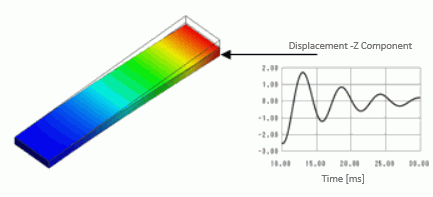General

• A cantilever is mechanically loaded. After it is unloaded, it starts to vibrate. The vibrations decays over time.

• The displacement and the mechanical stress are solved for each time step.

• Unless specified in the list below, the default conditions will be applied.

•

Analysis Space

 Item Settings Analysis Space 3D Model unit mm

Analysis Conditions

 Item Settings Solvers Mechanical Stress Analysis [Galileo] Analysis Type Transient analysis

The transient analysis is set up as follows.

Tab

Setting Item

Settings

Transient Analysis Tab

Table

 Calculation steps Output steps Time step [s] 5 1 0.002 100 1 0.0002

Rayleigh Damping Coefficients

Beta=0.0002

The load gradually increases in the first 5 steps. In the next 100 steps,

it is unloaded, and the damping vibration occurs.

0.01 – 0.03 [sec]: Vibration period

Model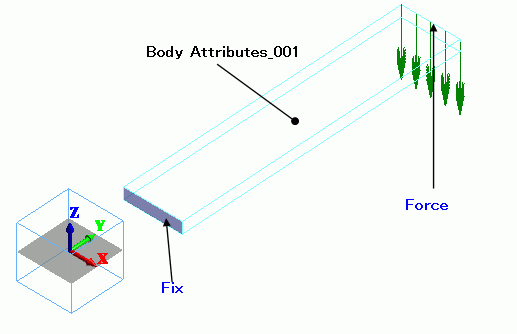Body Attributes and Materials

 Body Number/Type Body Attribute Name Material Name 0/Sheet Body_Attribute_001 002_Polycarbonate(PC)

Boundary Conditions

Boundary Condition Name/Topology

Tab

Boundary Condition Type

Settings

Fix/Face

Mechanical

Displacement

Select all X/Y/Z components.

UX=0, UY=0, UZ=0

Force/Face

Mechanical

X=0, Y=0, Z=-0.5×10^5

Time Dependency: Yes

 Time Weight 0 0 0.01 1 0.101 0 10 0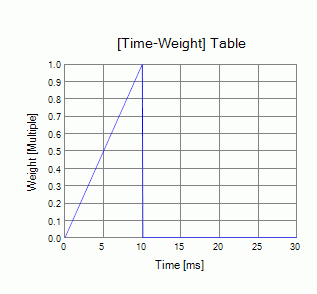Tips on Analysis Conditions and Boundary Conditions

Perform a resonant analysis and obtain the adequate time steps for the expected vibration period.

Results

The displacement diagram below shows the deformation at 0.01[sec] (Mode number 5). The color gradation contour indicates the Z displacement.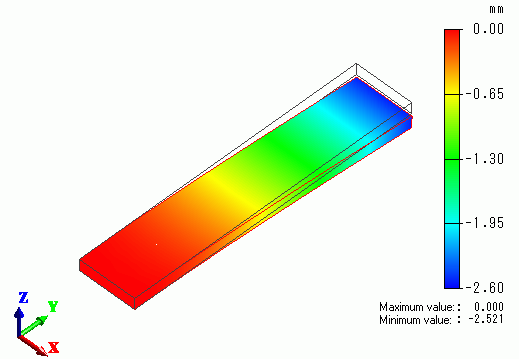Plotted below is the Z displacement of the cantilever tip in 0.01 to 0.03 [sec] (Mode numbers 5 through 105)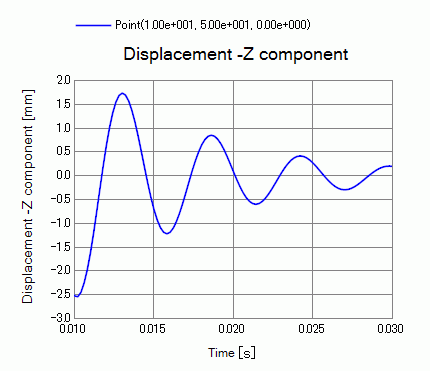The vibration decays as the time passes.

The figure below shows the vibration when Beta is set to 0.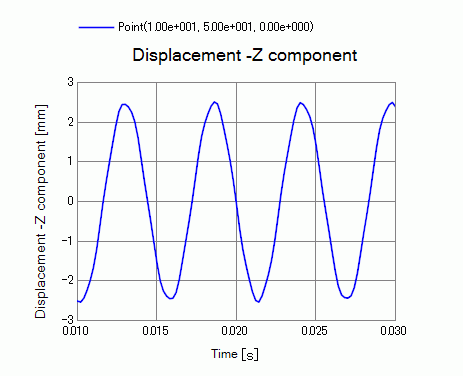There is no decay.

﻿